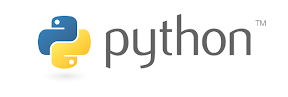# What is Python?

Posted on in programmingPython is a general-purpose, high-level programming language. It is one of the most popular programming languages in the world, used for a wide variety of tasks, including:

• Web development
• Data science
• Machine learning
• Artificial intelligence
• Software development
• Scientific computing
• Game development

Python is known for its simple, easy-to-learn syntax, which makes it a good language for beginners. It is also a very powerful language, capable of handling complex tasks.

Here's a quick Python example:

``````def fibonacci(n):
if n == 0:
return 0
elif n == 1:
return 1
else:
return fibonacci(n - 1) + fibonacci(n - 2)

for i in range(10):
print(fibonacci(i))
``````

This code defines a function called `fibonacci()` that calculates the Fibonacci sequence. The Fibonacci sequence is a series of numbers where each number is the sum of the two previous numbers. The first two numbers in the sequence are 0 and 1, so the output of the code is:

``````0
1
1
2
3
5
8
13
21
34
``````

The code first checks if the input number (n) is 0 or 1. If it is, then the function returns 0 or 1, respectively. Otherwise, the function recursively calls itself to calculate the Fibonacci numbers for n-1 and n-2, and then adds those two numbers together.

Python is a great language for beginners and experienced programmers alike. It is easy to learn, powerful, and versatile. If you are interested in learning Python, there are many resources available online and in libraries.

Here are some of the benefits of learning Python:

• It is a general-purpose language, so you can use it for a wide variety of tasks.
• It is easy to learn, with a simple syntax that is similar to English.
• It is a powerful language, capable of handling complex tasks.
• There are many resources available to help you learn Python, including online tutorials, books, and courses.

If you are interested in learning Python, I recommend checking out the following resources:

I hope this helps!